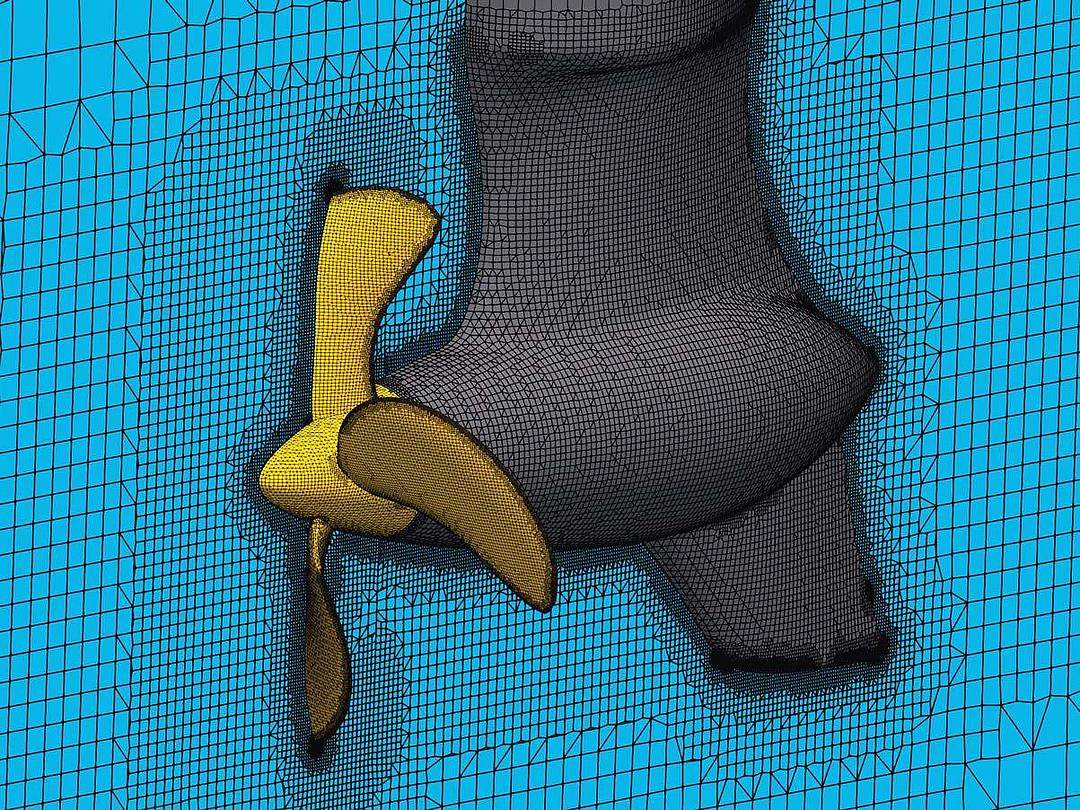# MODEL TRIAL 4.0: AN INSIGHT INTO CFD

Flows are complex physical processes and require special investigation methods. CFD simulations are used for this. Thanks to this computer-based method, SCHOTTEL customers benefit from even greater expertise in the design of their products

At SCHOTTEL, CFD simulations have been an integral part of the hydrodynamic design process for years. Computational Fluid Dynamics (CFD for short) can be used to simulate and analyze a wide range of different applications, such as open-water propeller performance, vessel resistance, towing power, manoeuvrability, risk of cavitation or noise development.

The findings obtained during a CFD simulation are then used for further optimization of the propulsion solutions.

### CONSERVATION EQUATIONS AS COMPUTATIONAL BASIS

In order to ensure that SCHOTTEL products are always state-of-the-art, detailed knowledge of their flow behaviour is a basic requirement. Until now, the main method for determining measured variables, such as pressure, thrust or speed, was model trials; the variables were then scaled up to full size. Although Computational Fluid Dynamics has been widely applied in research for many years, it has only recently gained greater recognition in the industrial environment. At SCHOTTEL, investments in the six-digit euro range have currently being made  to ensure that the high level of the CFD calculations is also maintained in the future.

Conservation equations for physical variables such as mass, momentum and energy, are used to describe the flow properties of complex components. At the end of the calculation, the computational solution of these equations provides exact information about the three-dimensional flow field throughout the entire area under investigation.

### IMPROVED UNDERSTANDING OF THE PHENOMENON

Even if CFD simulations are still associated with above-average expertise, high time requirements and powerful computers, they are generally considered to be the less expensive and faster alternative to complex model trials. CFD calculations benefit from the fact that the model can be freely expanded, scaled and modified at any time and with minimal effort. In this way, the simulations can also be applied to flows for which experiments are difficult to implement or empirical measurements cannot be determined.Example of a mesh for the CFD simulation of an SRE®: the flow area (blue) and surface mesh of the propeller (bronze) and the housing (grey) are shown.

## CONSERVATION EQUATIONS

Conservation equations are equations in which the value of a variable does not change, i. e. is conserved, in certain physical processes. The best-known law of conservation is that of the conservation of energy. Put colloquially: “The energy you put in is the same as the energy you get back. In other words, no energy is lost and no energy is created out of nothing.” The five conservation equations of mass, momentum in all dimensions and energy are also referred to as the Navier- Stokes equations.### CFD SIMULATION PROCEDURE

The procedure during a CFD simulation can be divided into three steps: pre-processing, the actual calculation during the solution phase and post-processing; in the latter step, the results are validated and visualized.

First of all, a 3D model is mapped: geometry details that are not, or only partially, relevant for the simulation are completely removed or represented in a simplified form. Following geo- metric preparation of the model, its so-called mesh is generated, in which the flow area is divided into a finite number of cells. A mesh of poor quality – for example one for which too few cells were generated, resulting in a mesh that is too coarse or contains strongly degenerated cells – can lead to significant errors in the result, even including failure of the simulation. In the next step (solution), calculation of the conservation equations is performed. Pre-processing also includes definition of the initial and boundary conditions, such as velocity, volume flow, pressure or speed. Correct definition of these parameters has considerable influence on the quality and accuracy of the results.

Pre-processing is followed by the actual calculation, which may take several hours or even days. This requires appropriate hardware and software that solves the conservation equations in the calculation area cell by cell.

### VALIDATION AND VISUALIZATION

Following successful calculation, the results  of computational simulations are checked  for plausibility. The validation process includes comparisons with empirical values, basic equations or values from model trials. In the final step, the results are visualized and presented in graphics, diagrams or animations.

Computational methods such as CFD not only combine state-of-the-art technologies with know-how acquired over many years, but also make a significant contribution to the successful development and production of efficient and reliable propulsion solutions.